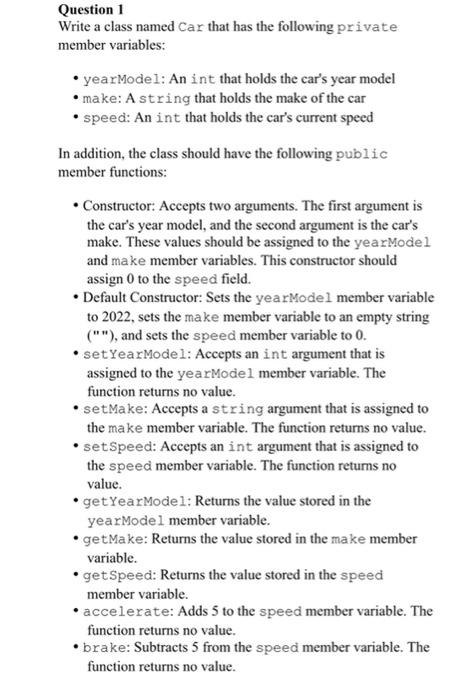# Question Solved1 Answer Question 1 Write a class named Car that has the following private member variables: - yearModel: An int that holds the car's year model - make: A string that holds the make of the car - speed: An int that holds the car's current speed In addition, the class should have the following public member functions: - Constructor: Accepts two arguments. The first Question 1 Write a class named Car that has the following private member variables: - yearModel: An int that holds the car's year model - make: A string that holds the make of the car - speed: An int that holds the car's current speed In addition, the class should have the following public member functions: - Constructor: Accepts two arguments. The first argument is the car's year model, and the second argument is the car's make. These values should be assigned to the yearModel and make member variables. This constructor should assign 0 to the speed field. - Default Constructor: Sets the yearModel member variable to 2022 , sets the make member variable to an empty string ("*), and sets the speed member variable to 0 . - setYearModel: Accepts an int argument that is assigned to the yearModel member variable. The function returns no value. - setmake: Accepts a string argument that is assigned to the make member variable. The function returns no value. - setSpeed: Accepts an int argument that is assigned to the speed member variable. The function returns no value. - getYearModel: Returns the value stored in the yearModel member variable. - getMake: Returns the value stored in the make member variable. - getSpeed: Returns the value stored in the speed member variable. - accelerate: Adds 5 to the speed member variable. The function returns no value. - brake: Subtracts 5 from the speed member variable. The function returns no value.80GQFL The Asker · Computer ScienceTranscribed Image Text: Question 1 Write a class named Car that has the following private member variables: - yearModel: An int that holds the car's year model - make: A string that holds the make of the car - speed: An int that holds the car's current speed In addition, the class should have the following public member functions: - Constructor: Accepts two arguments. The first argument is the car's year model, and the second argument is the car's make. These values should be assigned to the yearModel and make member variables. This constructor should assign 0 to the speed field. - Default Constructor: Sets the yearModel member variable to 2022 , sets the make member variable to an empty string ("*), and sets the speed member variable to 0 . - setYearModel: Accepts an int argument that is assigned to the yearModel member variable. The function returns no value. - setmake: Accepts a string argument that is assigned to the make member variable. The function returns no value. - setSpeed: Accepts an int argument that is assigned to the speed member variable. The function returns no value. - getYearModel: Returns the value stored in the yearModel member variable. - getMake: Returns the value stored in the make member variable. - getSpeed: Returns the value stored in the speed member variable. - accelerate: Adds 5 to the speed member variable. The function returns no value. - brake: Subtracts 5 from the speed member variable. The function returns no value.
More
Transcribed Image Text: Question 1 Write a class named Car that has the following private member variables: - yearModel: An int that holds the car's year model - make: A string that holds the make of the car - speed: An int that holds the car's current speed In addition, the class should have the following public member functions: - Constructor: Accepts two arguments. The first argument is the car's year model, and the second argument is the car's make. These values should be assigned to the yearModel and make member variables. This constructor should assign 0 to the speed field. - Default Constructor: Sets the yearModel member variable to 2022 , sets the make member variable to an empty string ("*), and sets the speed member variable to 0 . - setYearModel: Accepts an int argument that is assigned to the yearModel member variable. The function returns no value. - setmake: Accepts a string argument that is assigned to the make member variable. The function returns no value. - setSpeed: Accepts an int argument that is assigned to the speed member variable. The function returns no value. - getYearModel: Returns the value stored in the yearModel member variable. - getMake: Returns the value stored in the make member variable. - getSpeed: Returns the value stored in the speed member variable. - accelerate: Adds 5 to the speed member variable. The function returns no value. - brake: Subtracts 5 from the speed member variable. The function returns no value.